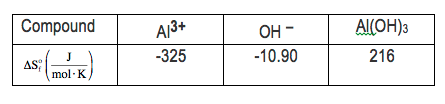Ch.6 - Chemical EquilibriumWorksheetSee all chapters

# Chemical Thermodynamics: Entropy

See all sections
Sections
The Equilibrium State
The Reaction Quotient
Le Chatelier's Principle
Chemical Thermodynamics: Enthalpy
Chemical Thermodynamics: Entropy
Chemical Thermodynamics: Gibbs Free Energy
Solubilty Product Constant
Protic Acids and Bases
The pH Scale
Acid Strength

Entropy represents the quantity of a system's thermal energy that couldn't be converted into mechanical work.

###### Understanding Entropy

Concept #1: Entropy represents the amount of disorder, chaos or randomness in our system.

Concept #2: As the distance between molecules increases there will be an increase in entropy.

Concept #3: When comparing the entropy of ionic compounds it is best to examine their lattice energies.

###### Entropy Calculations

Example #1: Predict the sign of entropy for each of the following processes?

a) Iat 90oC and 5.0 atm  →  I2 at 50oC and 10.0 atm

b) NH4Cl(s) →  HCl(g) +  NH3(g)

c) CH4(g)  +  2 O2(g)  →   CO2(g)  +  2 H2O(l)

Example #2: When an aqueous solution containing Al3+ at 25°C is mixed with an aqueous solution of hydroxide at 25°C an immediate precipitate of insoluble aluminum hydroxide is formed:

Al3+ (aq) + 3 OH – (aq)  →  Al(OH)3 (s)Practice: Which of the following has the greatest entropy, S?